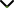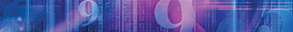# Fractals and Fractional Calculus in Mathematical Physics

Publishing date
01 Feb 2023
Status
Closed
07 Oct 2022

Guest Editors

1Yibin University, Yibin, China

2Guizhou University, Guiyang, China

3Institute of Actuarial Science and Data Analytics, UCSI University, Kuala Lumpur, Malaysia

This issue is now closed for submissions.

# Fractals and Fractional Calculus in Mathematical Physics

This issue is now closed for submissions.

## DescriptionFractal theory is a compact branch of nonlinear science and has significant applications in porous media, aquifers, turbulence, and other media which usually exhibit fractal properties. Fractional calculus has great importance where all the elements can be found such as the idea of fractional-order integration and differentiation, and the mutually inverse relationship between them. Fractional calculus has its applications in miscellaneous fields of engineering and science such as electromagnetics, viscoelasticity, fluid mechanics, electrochemistry, biological population models, optics, and signal processing.

The theory and applications of fractional calculus greatly prolonged over the 19th and 20th centuries, and numerous contributors have given definitions for fractional derivatives and integrals. Mathematical research on fractals has now reached such a level, where beautiful concepts of fractional calculus are developed in direct contact with engineering concerns. Numerous analytical and numerical investigations have played a decisive role in particular areas but there are still many challenges to find out the exact solution.

This Special Issue aims to collect ideas and significant contributions to the theories and applications of analytic inequalities, functional equations, and differential equations involving fractals and fractional calculus including theoretical and numerical features in future directions. We welcome original research and review articles.

Potential topics include but are not limited to the following:

• The two-scale fractal derivative
• Fractal geometry
• Applications of fractal theory in science and engineering
• Analytical and numerical solution of fractional differential equations
• Fractal and fractional calculus
• Fractal derivative and fractal space
• Variational theory and variational principle
• Fractal semi-inverse method
• New numerical schemes for fractal-fractional operators
• Fractal-fractional calculus
• Applications of fractional calculus in mathematical physics
• Fractional differential equations in fractal mediaJournal metrics
See full report
Acceptance rate21%
Submission to final decision79 days
Acceptance to publication21 days
CiteScore1.900
Journal Citation Indicator0.430
Impact Factor1.2Author guidelinesEditorial boardDatabases and indexing

Article of the Year Award: Impactful research contributions of 2022, as selected by our Chief Editors. Discover the winning articles.